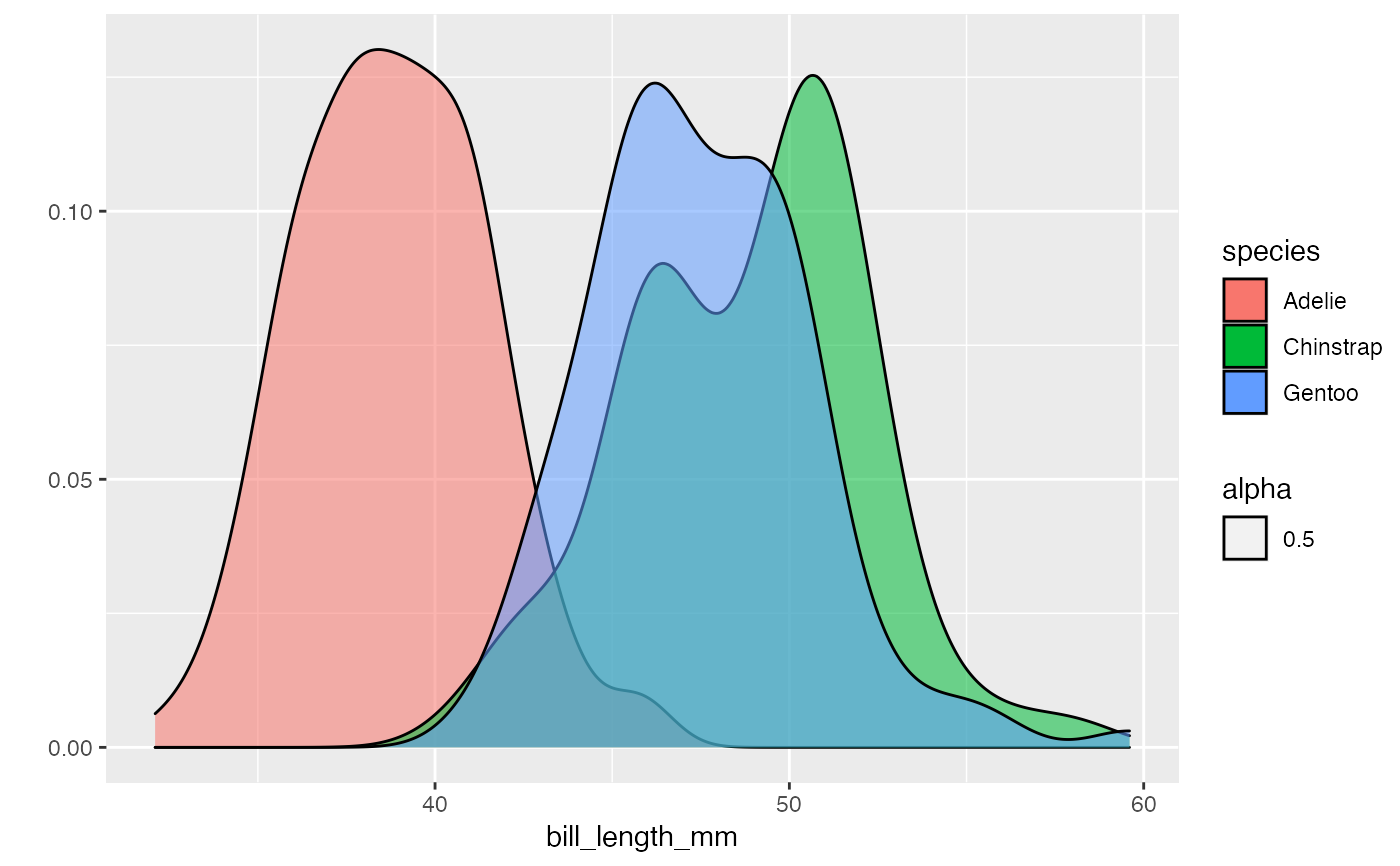The qplot() function is traditionally used when your data is not stored in a data.frame/tibble and are "loose" within your environment and can be convenient if you are familiar with the base plot(), but haven't quite learned the grammar of ggplot2. It is a wrapper that creates a ggplot() style plot.

However, creating a ggplot() object can be complex and inconvenient, especially if you want a simple plot (e.g. a basic histogram). It also requires you to either break out of a pipeline, or ensure your ggplot() functions are at the end of a pipe (or introduce curly braces {} to your pipeline).

Therefore, the pipe_qplot() function will run a qplot() function for it's side effects and return your original input unchanged. It can also save your plot if needed.

pipe_qplot(
data,
x,
y,
...,
facets = NULL,
margins = FALSE,
geom = "auto",
xlim = c(NA, NA),
ylim = c(NA, NA),
log = "",
main = NULL,
xlab = NULL,
ylab = NULL,
asp = NA,
stat = stat,
position = position,
theme = NULL,
save.options = NULL,
print.plot = T
)

## Arguments

data the data being passed through the pipeline aesthetic arguments to be passed to the qplot() function see the qplot() documentation for more information regarding these arguments. a character string that links to a function of the form theme_*(), e.g. the agrument theme="bw" will apply theme_bw() list of values to be passed to ggsave() (if NULL, plot won't be saved) should the plot be displayed? Should only be used if save.options is not NULL

## Examples


palmerpenguins::penguins %>%
dplyr::group_by(species) %>%
pipe_qplot(bill_length_mm,
fill = species,
theme = "light",
geom = "density",
alpha = 0.5,
binwidth = 0.1
) %>%
dplyr::summarise(mean = mean(bill_length_mm))
#> Warning: Ignoring unknown parameters: binwidth#> Warning: Removed 2 rows containing non-finite values (stat_density).#> summarise() ungrouping output (override with .groups argument)#> # A tibble: 3 x 2
#>   species    mean
#>   <fct>     <dbl>
#> 3 Gentoo     NA